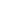FLAT 40% OFF

Ends in

# CBSE 12th Chemistry Preparation Tips and Sample Paper 2020

Published 7 months agoCBSE will conduct the board examinations for XIIth students in February-March 2020. CBSE will release the Board Exam 2020 Date Sheet by the end of December 2019. Students can check some of the important points related to exam for preparation

CBSE Class 12th Chemistry Board Exam Paper Pattern 2020:

The CBSE Class 12th Chemistry paper is of 3-hour duration and the theory exam is of 70 marks while practical is of 30 marks.

The candidates are required to answer all questions given in the question paper. The Class 12th Chemistry question paper have four sections - A, B, C, and D.
• Section A: In this section, there are 20 very short answer questions (objective type) and each carry 1 mark
• Section B: There will be seven short answer questions in this section and each will carry 2 marks
• Section C: There will be six long answer questions in this section carrying 3 marks each
• Section D: There will be 3 long answer questions in this section carrying 5 marks each

The students are allowed to use log tables if necessary, however, use of calculators is forbidden.

Preparation Tips for CBSE Class 12th Chemistry
1) Read the complete syllabus: The NCERT book is the best study material for board exam preparation. Therefore, pay attention to each chapter and aim to understand all the important equations and concepts.

2) Attention more on main topics: Some of the most important chapters for Chemistry paper are - Physical Chemistry, Organic Chemistry, Inorganic Chemistry, p-block elements, d- and f- block elements, Coordination Compound, Metallurgy etc.

3) Solve previous year and sample test papers: Students are advised to practice previous year board exam question papers this will give you an idea about the type of questions asked and setting a time limit will improve your speed. Mock tests are also important to check preparation for board exam.

Download free previous years and sample papers for CBSE class 12th preparation.

(from:timesofindia.indiatimes.com)